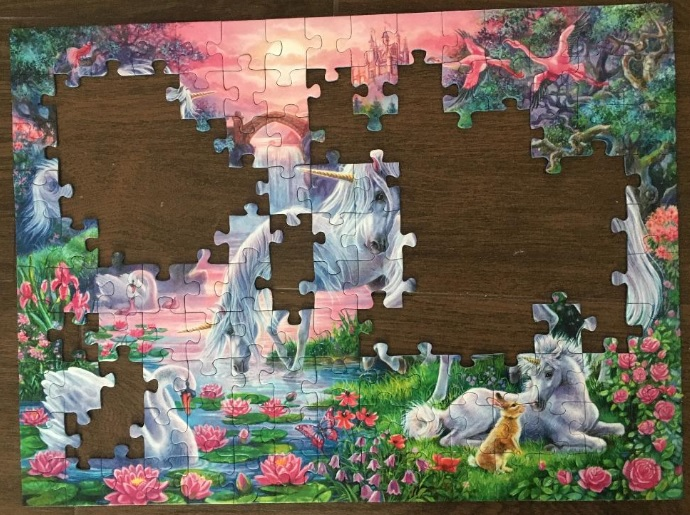# 1491 How Many Square Units Do You See in This Partially Completed Puzzle?

Contents

### Today’s Puzzle:

My grandchildren had put this puzzle together many times on their own but wanted me to help them this time. The picture on the box was very helpful and let me know there were four unicorns, but I could only find puzzle pieces for three horns instead of four. I found the missing horn under some furniture in the living room, but I still wondered if any other puzzle pieces had been misplaced. I used multiplication, addition, and subtraction to figure out how many puzzle pieces still needed to be placed on this partially completed puzzle. Can you also figure out the number of puzzle pieces that still need to be placed?I counted the number of puzzle pieces in the box and determined that two pieces were missing. Knowing that two puzzle pieces are missing, can you tell me how many more puzzle pieces are in the box waiting to be placed on the puzzle?

Each puzzle piece is approximately equal to one square unit. If you can determine the area of the entire puzzle and the area of the missing pieces, then you can determine the area of the incomplete puzzle pictured above. Go ahead, give it a try!

### Factors of 1491:

• 1491 is a composite number.
• Prime factorization: 1491 = 3 × 7 × 71.
• 1491 has no exponents greater than 1 in its prime factorization, so √1491 cannot be simplified.
• The exponents in the prime factorization are 1, 1, and 1. Adding one to each exponent and multiplying we get (1 + 1)(1 + 1)(1 + 1) = 2 × 2 × 2 = 8. Therefore 1491 has exactly 8 factors.
• The factors of 1491 are outlined with their factor pair partners in the graphic below.### Another Fact about the number 1491:

1491 is the 21st nonagonal number because
21(7·21 – 5)/2 = 1491.

This site uses Akismet to reduce spam. Learn how your comment data is processed.